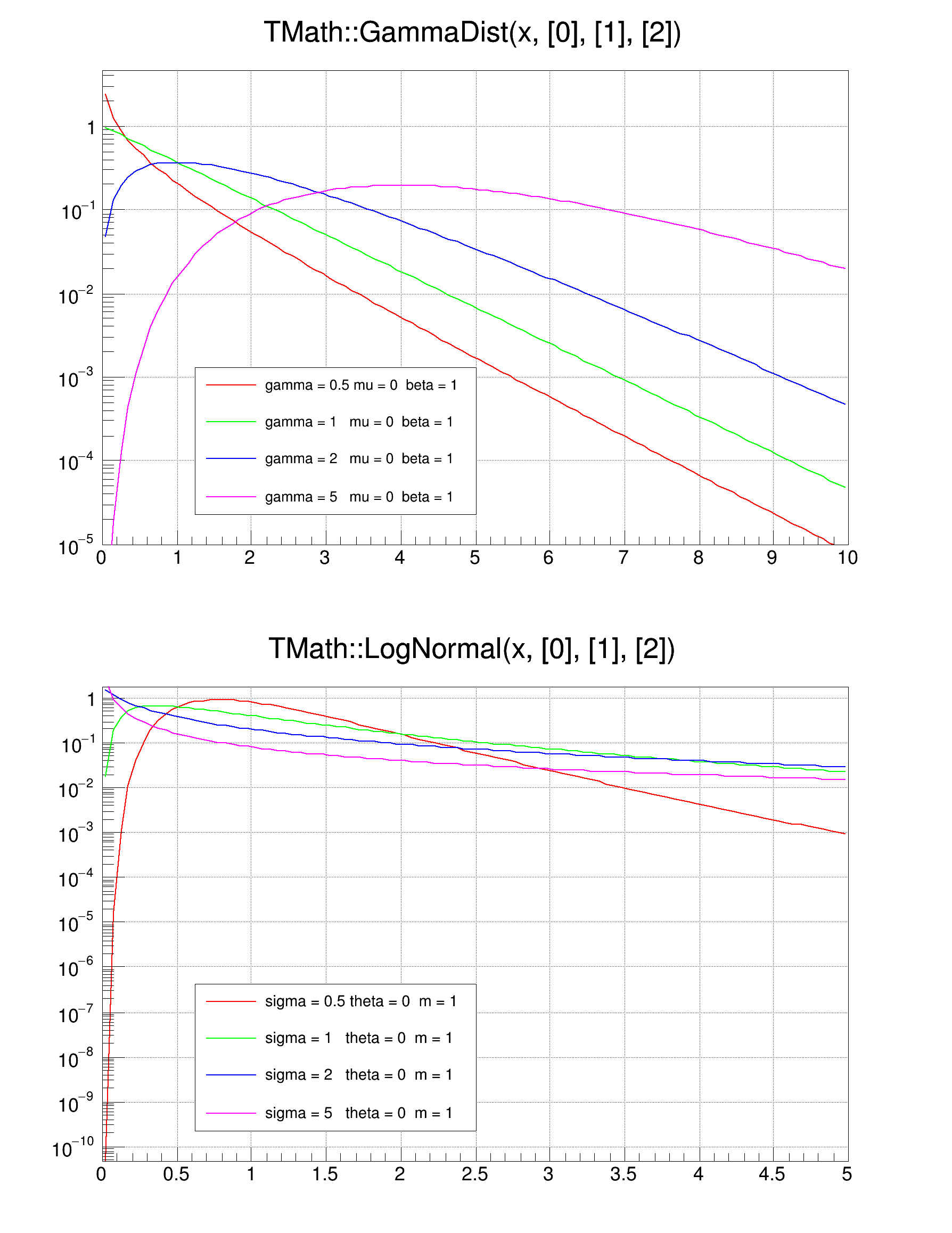ROOT   Reference Guide
Searching...
No Matches
mathGammaNormal.C File Reference

## Detailed Descriptionvoid mathGammaNormal(){
TCanvas *myc = new TCanvas("c1","gamma and lognormal",10,10,600,800);
myc->Divide(1,2);
TF1 *fgamma = new TF1("fgamma", "TMath::GammaDist(x, , , )", 0, 10);
fgamma->SetParameters(0.5, 0, 1);
TF1 *f1 = fgamma->DrawCopy();
f1->SetMinimum(1e-5);
fgamma->SetParameters(1, 0, 1);
TF1 *f2 = fgamma->DrawCopy("same");
fgamma->SetParameters(2, 0, 1);
TF1 *f3 = fgamma->DrawCopy("same");
fgamma->SetParameters(5, 0, 1);
TF1 *f4 = fgamma->DrawCopy("same");
TLegend *legend1 = new TLegend(.2,.15,.5,.4);
legend1->AddEntry(f1,"gamma = 0.5 mu = 0 beta = 1","l");
legend1->AddEntry(f2,"gamma = 1 mu = 0 beta = 1","l");
legend1->AddEntry(f3,"gamma = 2 mu = 0 beta = 1","l");
legend1->AddEntry(f4,"gamma = 5 mu = 0 beta = 1","l");
legend1->Draw();
//TMath::LogNormal
TF1 *flog = new TF1("flog", "TMath::LogNormal(x, , , )", 0, 5);
flog->SetParameters(0.5, 0, 1);
TF1 *g1 = flog->DrawCopy();
flog->SetParameters(1, 0, 1);
TF1 *g2 = flog->DrawCopy("same");
flog->SetParameters(2, 0, 1);
TF1 *g3 = flog->DrawCopy("same");
flog->SetParameters(5, 0, 1);
TF1 *g4 = flog->DrawCopy("same");
TLegend *legend2 = new TLegend(.2,.15,.5,.4);
legend2->AddEntry(g1,"sigma = 0.5 theta = 0 m = 1","l");
legend2->AddEntry(g2,"sigma = 1 theta = 0 m = 1","l");
legend2->AddEntry(g3,"sigma = 2 theta = 0 m = 1","l");
legend2->AddEntry(g4,"sigma = 5 theta = 0 m = 1","l");
legend2->Draw();
}
#define e(i)
Definition RSha256.hxx:103
@ kRed
Definition Rtypes.h:66
@ kGreen
Definition Rtypes.h:66
@ kMagenta
Definition Rtypes.h:66
@ kBlue
Definition Rtypes.h:66
virtual void SetLineColor(Color_t lcolor)
Set the line color.
Definition TAttLine.h:40
The Canvas class.
Definition TCanvas.h:23
Definition TCanvas.cxx:708
1-Dim function class
Definition TF1.h:213
virtual void SetMinimum(Double_t minimum=-1111)
Set the minimum value along Y for this function In case the function is already drawn,...
Definition TF1.cxx:3420
virtual TF1 * DrawCopy(Option_t *option="") const
Draw a copy of this function with its current attributes.
Definition TF1.cxx:1352
virtual void SetParameters(const Double_t *params)
Definition TF1.h:644
This class displays a legend box (TPaveText) containing several legend entries.
Definition TLegend.h:23
TLegendEntry * AddEntry(const TObject *obj, const char *label="", Option_t *option="lpf")
Add a new entry to this legend.
Definition TLegend.cxx:330
virtual void Draw(Option_t *option="")
Draw this legend with its current attributes.
Definition TLegend.cxx:423
The most important graphics class in the ROOT system.